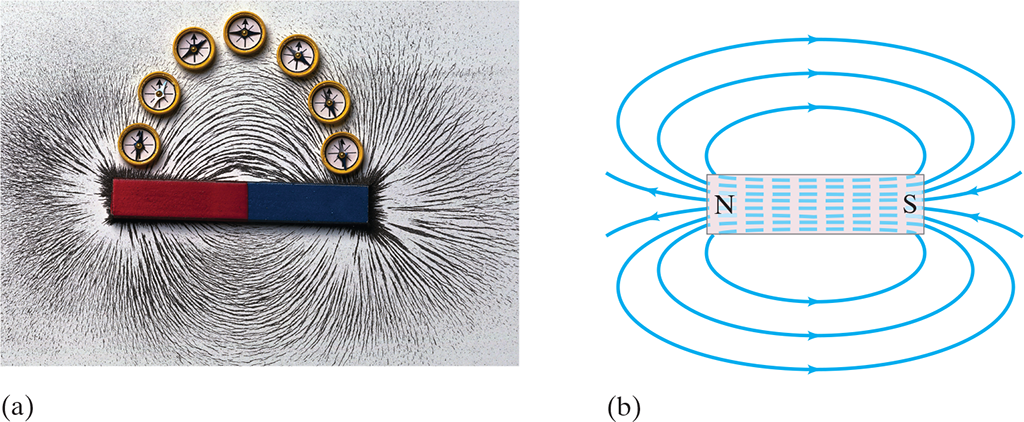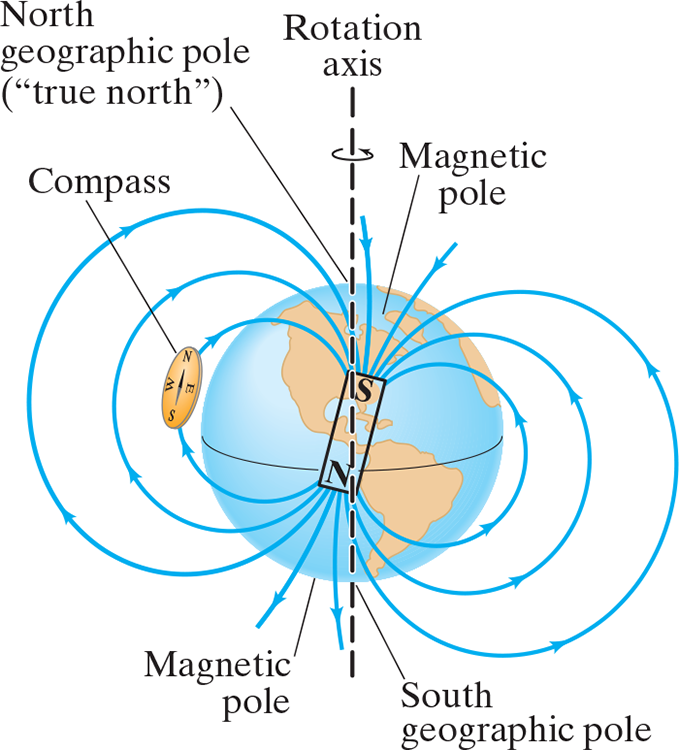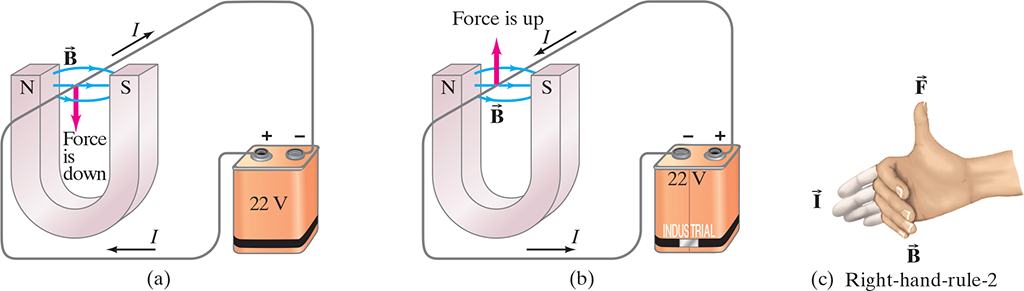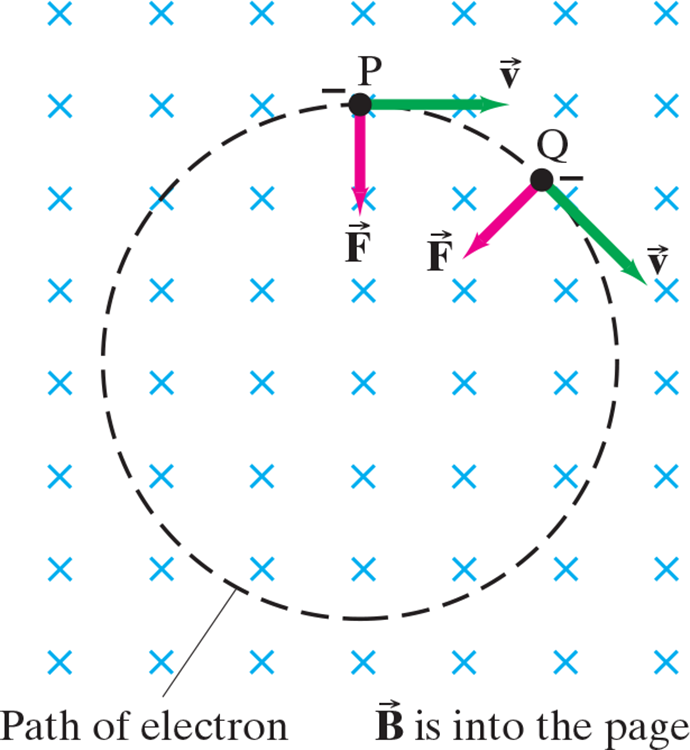27: Magnetism

# 27.1 Magnets and Magnetic Fields #

• Every magnet has two ends or faces called poles which are where the magnetic field is strongest
• If a magnet is suspended so it can move freely, one pole will point north
• Aptly, this side is called the north pole
• Magnetic poles aren’t like electric charge: Positive or negative charge can easily be isolated, but we can never isolate a magnetic pole
• That is, if you cut a magnet is half you don’t obtain isolated north and south poles.
• Rather, you end up with two new magnets each with north and south poles
• Ferromagnetic: Materials with a strong magnetic effect i.e. iron, cobalt, nickel, gadolinium
• Similar to how we picture electric fields around a charge, we can picture magnetic fields surround a magnet
• Field lines should be drawn so that (1) the direction of the magnetic field is always tangent to a field line everywhere and (2) the number of lines per unit area is proportional to the magnetic field strength(a) Visualizing magnetic field lines around a bar magnet, using iron filings and compass needles. The red end of the bar magnet is its north pole. The N pole of a nearby compass needle points away from the north pole of the magnet. (b) Diagram of magnetic field lines for a bar magnet.

## Earth’s Magnetic Field #

• Earth’s magnetic poles are not exactly through the geographic pole (axis of rotation)
• The angular difference between the direction of the compass needle (which points along the magnetic field lines) at any location and true (geographical) north varies between .$0 - 20^\circ$ with location
• Earth’s magnetic field at most location is not tangent to earth’s surface
• Angle of Dip: The angle that Earth’s magnetic field makes with the horizontal at any pointThe Earth acts like a huge magnet. But its magnetic poles are not at the geographic poles (on the Earth’s rotation axis).

# 27.2 Electric Currents Produce Magnet Fields #

(a) Deflection of compass needles near a current-carrying wire, showing the presence and direction of the magnetic field. (b) Iron filings also align along the direction of the magnetic field lines near a straight current-carrying wire. (c) Diagram of the magnetic field lines around an electric current in a straight wire. (d) Right-hand-rule-1 for remembering the direction of the magnetic field: when the thumb points in the direction of the conventional current, the fingers wrapped around the wire point in the direction of the magnetic field. (.$\vec B$ is the symbol for magnetic field).

# 27.3 Force on an Electric Current in a Magnetic Field #

• By Newton’s third law, we can see that a magnet exerts a force on a current-carrying wire
• The direction of the force is always perpendicular to the direction of the current and also perpendicular to the direction of the magnetic field .$\vec B$
• Use right hand rule!$$dF_\vec{B} = dq (\vec v \times \vec B) = dq\bigg(\frac{d\vec l}{dt}\times \vec B\bigg) = I (d\vec l \times \vec B)$$ $$\dots\ \vec F = I (\vec l \times \vec B) = I l b \sin\theta$$
• .$\vec l$ is the vector whose magnitude is the length of the wire its direction is along the (straight) wire in the direction of the conventional (positive) current
• We use the last equation if .$\vec B$ isn’t uniform or if the wire doesn’t form angle .$\theta$ with .$\vec B$ everywhere

# 27.4 Force on an Electric Charge Moving in a Magnetic Field #

• Recall, .$N$ particles, each charge .$q$, pass by a given point in time .$t$, they constitute current .$I = N q/t$
• Lets say in .$t$ time, a particle charge .$q$ moves distance .$l$ in a magnetic field .$\vec B$
• We know from kinematics that .$\vec l = \vec v t$ where .$\vec v$ is the velocity of the particle
• Using the 27.3 equation, we can find the force on all of these .$N$ particles as $$\vec F = I\vec l \times \vec B = (Nq/t)(\vec v t) \times \vec B = Nq\vec v \times \vec B$$
• Thus, the force on just one of the .$N$ particles is $$\vec F = q \vec v \times \vec B = qvB \sin\theta$$
• Realize that we can save a lot of pain if we know that the magnetic field is uniform in which case .$\vec F_\vec{B} = 0$ because the forces on opposite segments (sides) cancel out

## Uniform Field Path #Force exerted by a uniform magnetic field on a moving charged particle (in this case, an electron) produces a circular path.

• Notice the field goes into the paper, denoted with .$\times$
• Because force is always orthogonal to .$\vec v$, the magnitude of .$\vec v$
• The centripetal acceleration has magnitude .$a = v^2/r$
• Thus we can derive $$F = ma \Longrightarrow qvB = m \frac{v^2}{r}$$ $$\dots \Longrightarrow r = \frac{mv}{qB}$$
• The time .$T$ it takes a particle with charge .$q$ and speed .$v$ to make a revolution is $$T = \frac{2\pi\cdot r}{v} = \frac{2\pi m}{qB}$$ $$f = \frac{1}{T} = \frac{qB}{2\pi m}$$

## Problem Solving #

Magnetic fields are somewhat analogous to the electric fields, but there are several important differences to recall:

1. The force experienced by a charged particle moving in a magnetic field is orthogonal to the direction of the magnetic field (and to the direction of the velocity of the particle), whereas the force exerted by an electric field is parallel to the direction of the field (and independent of the velocity of the particle).
2. The right hand rule, in its different forms, is intended to help you determine the direction of magnetic field, and the force a field exerts, and/or the directions of electric current or charged particle velocity. The right-hand rules to the right are designed to deal with the “perpendicular” nature of these quantities.

## With Electric Field #

• Lorentz Equation: A particle charge .$q$ moving with velocity .$\vec v$ in the presence of both a magnetic field .$\vec B$ and electric field .$\vec E$ experiences a force $$\vec F = q (\vec E + \vec v \times \vec B)$$
• Realize that the magnetic field cannot alter speed (do work), it can only alter the direction!
• To change an objects speed, you must apply a force along the objects direction of motion
• The magnetic field exerts a force on particles moving orthogonal to it
• Therefore, no work can be done because the particle can only move orthogonal to the magnetic field
• That being said, realize that this field is responsible for the circular, constant speed, motion
• This is why earth’s magnetic field deflects, but doesn’t slow down, charged particles from outer space

# 27.5 Torque on a Current Loop; Magnetic Dipole Moment #

Calculating the torque on a current loop in a magnetic field .$\vec B$

(a) Loop face parallel to .$\vec B$ field lines; (b) top view; (c) loop makes an angle to .$\vec B$, reducing the torque since the lever arm is reduced. The vector .$\vec \mu$ is the “magnetic moment”.

• When an electric current flows in a closed wire loop that’s in an external magnetic field, the magnetic force on the current can produce a torque $$\tau = I aB \frac{b}{2} + I aB \frac{b}{2}$$ $$\dots = IabB$$
• .$A = ab$ is the area of the loop
• .$B$ is scalar of the magnetic field
• Notice, the vertical (orthogonal) sections of wire experience no force from the magnetic field
• If we have a coil of .$N$ loops of wire, the current is then .$NI$ so torque becomes $$\tau = NIAB$$
• We call .$\vec \mu = NI \vec A$ the magnetic dipole moment
• The direction of .$\vec A$ (and thus .$\vec \mu$) is defined as perpendicular to the plane of the coil
• We can then re-write our torque eq as $$\vec \tau = NI \vec A \times \vec B$$ $$\dots \vec \tau = \vec \mu \times \vec B$$
• Dipoles have some potential energy, found by $$U = \int \tau d\theta = \int NIAB\sin\theta d \theta$$ $$\dots = -\mu B \cos\theta = - \vec \mu \cdot \vec B$$

# 27.8 Hall Effect #

• When a current-carrying conductor is held fixed in a magnetic field, the field exerts a sideways force on the charge moving in the conductor
• E.x, if electrons move to the right in the rectangular conductor, the inward magnetic field will exert a downward force: (a)
• This force is .$F_B = -e\vec v_d \times \vec B$
• .$v_d$ is drift velocity
• Thus, electrons tend to move towards side .$D$
• This creates a potential difference (called the Hall emf), creating field .$\vec E_H$
• This field exerts force .$e\vec E_H$ on the moving charge
• These forces are equal, that is, $$e E_H = ev_d B$$ $$\therefore E_H = v_d B$$
• Hall emf is then, asm uniform .$E_H$, $$\mathscr{E}_H = E_H d = v_d B d$$
• .$d$ is the width of the conductor

The Hall effect. (a) Negative charges moving to the right as the current. (b) Positive charges moving to the left as the current.

# 27.9 Mass Spectrometer #

• Mass spectrometers are used to measure the masses of atoms

• Steps:

1. Ions are produced (by a current or heating) and they pass through slit .$S_1$
2. Ions then pass through a region with perpendicular electric and magnetic fields.
• Here, .$F_E = qE$ is equal to .$F_B = qvB$
• Therefore, .$v = \frac{E}{B}$ for all ions that pass the slit into .$S_2$; the rest are deflected

1. Entering .$S_2$, there is only magnetic field .$B’$ so the ios follow a circular path
• Newtons second gives us .$F = ma \Longrightarrow qvB’ = mv^2/r$
• Since we know all terms, we can solve for mass: .$m = \frac{qB’r}{v} = \frac{qBB’r}{E}$